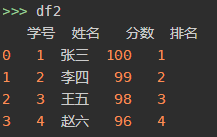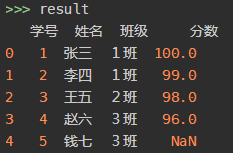# 使用 pandas 实现 excel 的 vlookup 功能

## １. 读取数据

``````import openpyxl
import pandas as pd
``````## ２. 实现 vlookup 功能

``````#只在table1添加分数列，不添加排名列
result = pd.merge(df1,df2.loc[:,['学号','分数']],how='left',on = '学号')
``````## ３. 保存结果

``````writer = pd.ExcelWriter('result.xlsx')
result.to_excel(writer,index=False)
writer.save()
``````